Next: Theory: Optimization Methods Up: Statistical Background Previous: Optimal Combination

## Error Propagation

In order to use a piece of information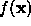derived from a set of measures x we must have information regarding its likely variation. If x has been obtained using a measurement system then we must be able to quantify measurement accuracy. Then we require a method for propagating likely errors on measurement through to. Assuming knowledge of error covariance this can be done as follows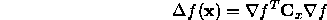The method simply uses the derivative of the function f as a linear approximation to that function. This is sufficient provided the expected variation in parameters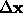is small compared to the range of linearity of the function. As an example we can take the Poisson distribution itself which for large numbers is expected to have a standard deviation of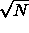where N is the mean of the distribution. We will call a sample random variable from this distribution s. If we now construct a new measure given by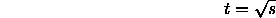then we can show, using a simplified form of error propagation for one parameter, that the expected variance on t is given by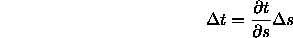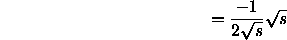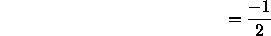Thus the distribution of the square-root of a random variable drawn from a Poisson distribution with large mean will be constant. This result will be used to generate the Matusita probability distribution comparison metric.

When the problem not permit algebraic manipulation in this form due to significant non-linear behaviour in the range of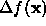or functional discontinuities then numerical approaches may be appropriate. These techniques are often referred to as Monte-Carlo approaches because they make use of random number generation techniques to generate sample distributions.

Bob Fisher
Fri Mar 28 14:12:50 GMT 1997